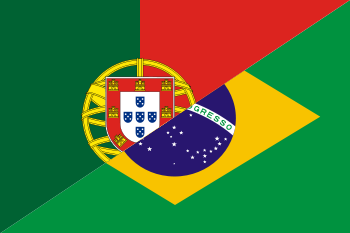# A5 Seminars

### IME - USPPortuguês

Welcome to the A5 Group Seminar page! This is a general-audience colloquium series for all members of the IME math community, our aim is to introduce research level math for undergraduate and gradute students at IME.

The lectures this term will take place every two weeks, on Tuesdays at 2 pm. A hybrid model will be adopted: when they are virtual, seminars will be broadcasted in some classroom at IME-USP.

To see the next lecture click here.

## Talks this term

March 29
Speaker
Pietro Mesquita Piccione
Title
A counterexample for the periodic orbit conjeture
Abstract
In this presentation, I will give a counterexample to the periodic orbit conjecture, which is: "There is no flow in a compact variety whose orbits are all closed and such that the length of the orbits is unbounded." The counterexample will be the flow of a vector field, in the product of the Heisenberg manifold by a 2-torus, which will result in a (real) analytic manifold of dimension 5. This presentation will be based on the article "A counterexample to the periodic orbit conjecture", by Dennis Sullivan, the most recent winner of the of the Abel Prize.
Time
2 pm
Model
Online lecture with broadcast
Recording
April 19th
Speaker
Claudio Gorodski
Title
The diameter of quotients of the sphere
Abstract
We consider an arbitrary quotient $$X = S^n(1)/G$$ of the unit sphere $$S^n(1)$$ by a group of isometries $$G$$ and show that the diameter of $$X$$ is zero or greater than a universal constant $$\epsilon \gt 0$$. What is new is the independence of $$\epsilon$$ from $$n$$. The classification of finite simple groups is used in this proof. (Joint work with C. Lange, A. Lytchak, and R. A. E. Mendes).
Time
2 pm
Model
On-site
Room
B-6
May 3rd
Speaker
Severino Toscano
Title
The Toeplitz Operator Index
Abstract

The final goal of the seminar is to give an outline of the proof of a formula for the Fredholm index of a Toeplitz operator on the circle with continuous symbol. I hope to be able to explain all the definitions and give a good idea of why the formula is true assuming only concepts of Measure Theory and Functional Analysis studied in the courses of our Bachelor's degree in mathematics. This "index theorem" for Toeplitz operators is the simplest of a series of results that express the Fredholm index of an operator (an analytic datum) in terms of some topological information carried by the operator. In this case, the Fredholm index is equal to minus the number of rotations of a continuous function wich doesn't vanish, used to define the Toeplitz operator in question.

This lecture will be a condensed version of two lectures I I gave in the course Panoramas da Matemática (MAT 554) in 2020.

Time
2 pm
Model
On-site
Room
B-9
May 17th
Speaker
João Ruiz
Title
Modular Forms: why should I care?
Abstract

In this talk we will explore a little bit of the theory of modular forms: how they arise in nature, how they can be used to solve Number Theory problems, and how we can place them in a more general context, with deep connections context, with deep connections to other areas of mathematics.

Highlights of the lecture include: "With how many squares can an integer be made?" and "A meeting of Number Theory and Algebraic Geometry"!

Time
2 pm
Model
Online lecture with broadcast
Recording
Room
B-9
May 31st
Speaker
Marcos Alexandrino (IME-USP)
Title
Parallel transport in curved spaces
Abstract

The concepts of connection and parallel transport are fundamental in Riemannian geometry and afford, among other things, interpretations of curvature.

This talk is aimed at a broad undergraduate mathematics audience and will be roughly divided into three parts. First we will introduce the concepts of connection, parallel transport, Holonomy groups, geodesics, and curvature. We will then cover Ambrose Singer's theorem.

Finally we will comment on relations between parallel transport and singular foliations, presenting results on recent research.

Time
2 pm
Model
On-site
Room
B-9
June 7th
Speaker
Ana Luiza Tenório (IME-USP)
Title
Sheaves in Opinion Dynamics
Abstract

In the article "Opinion Dynamics on Discourse Sheaves", published in 2021, Jakob Hansen and Robert Ghrist present how to use cellular sheaves and their respective cohomology theory to model the evolution of people's opinions/preferences over time. The main idea of the paper is to generalize the Laplacian matrix via the Laplacian sheaf in the context of graphs.

In this lecture I will introduce basic concepts of category theory, necessary for understanding the article, and discuss the main advantages of this approach such as the ability to identify the presence of people who lie.

Time
2 pm
Model
On-site
Recording
Slides
Room
B-9
June 28th
Speaker
Marcelo Moreira (IME-USP)
Title
Tilting Theory: an example in the Category of Modules
Abstract

In the area of Representation of Algebras, current research topics, such as tau-tilting theory and subcategory $$n$$-cluster-tilting, are inspired by tilting theory. This theory is based on the presence of a special module $$T$$ called the tilting module.

In this lecture, an example in the category of finitely generated modules over a $$C$$-algebra $$A$$ of finite dimension will be presented. The tilting theory shows an intimate connection between the algebras $$A$$ and $$B = \operatorname{End} T$$.

Time
2 pm
Model
On-site
Room
B-9
Contact
acinco [at] ime.usp.br
Organizers
Adriana Mayumi Shiguihara, Guilherme Sobreira, Henrique de Oliveira Rocha, Pietro Mesquita Piccione, Thiago Brevidelli, Thiago Guelfi & Victor Pirozelli